Miscellaneous

Chapter 9 Class 11 Sequences and Series
Serial order wise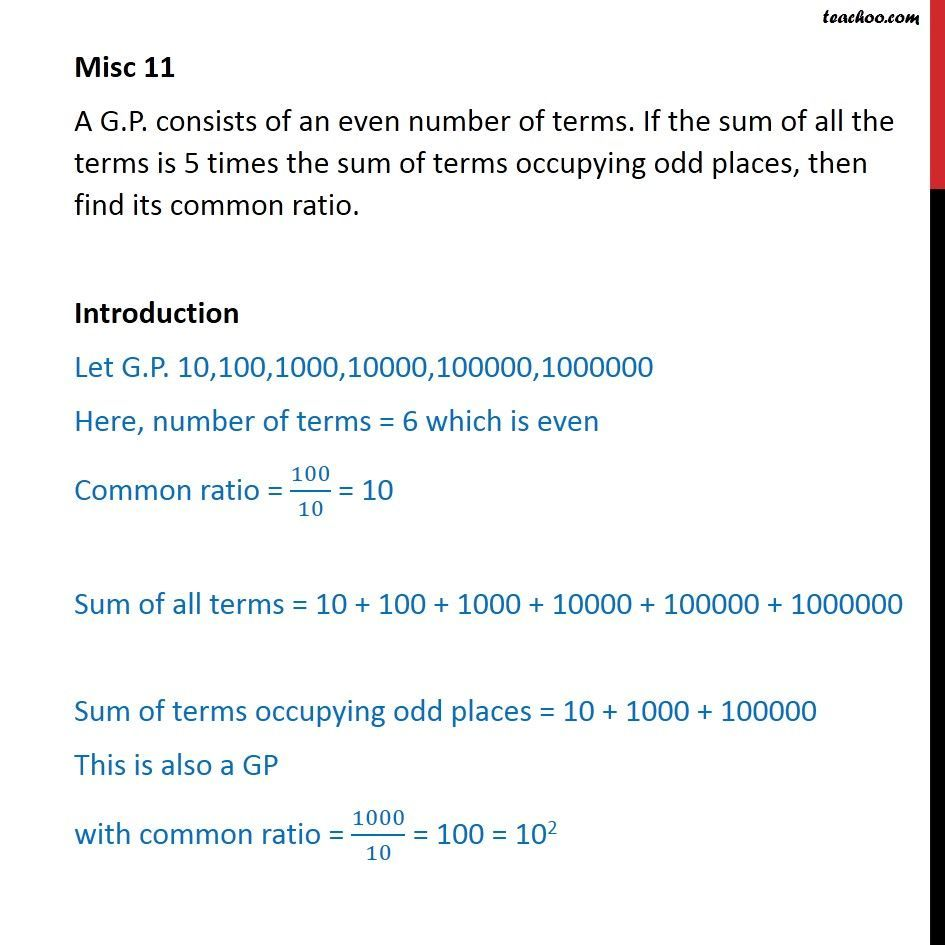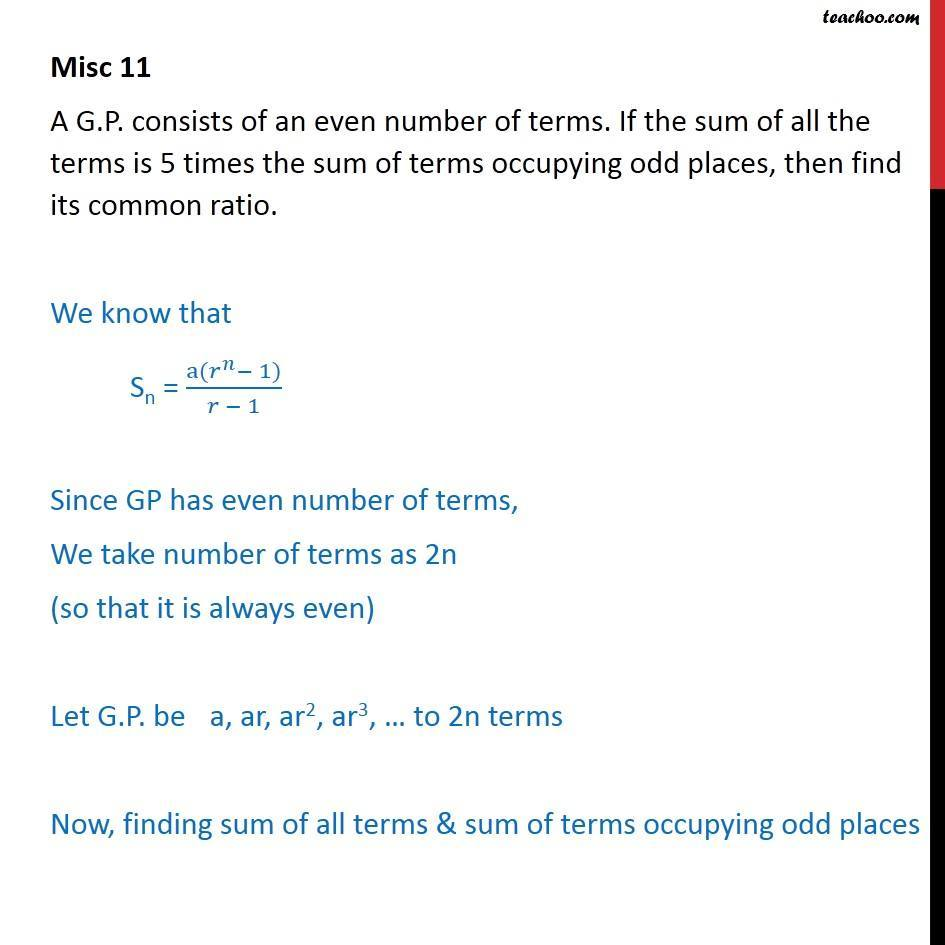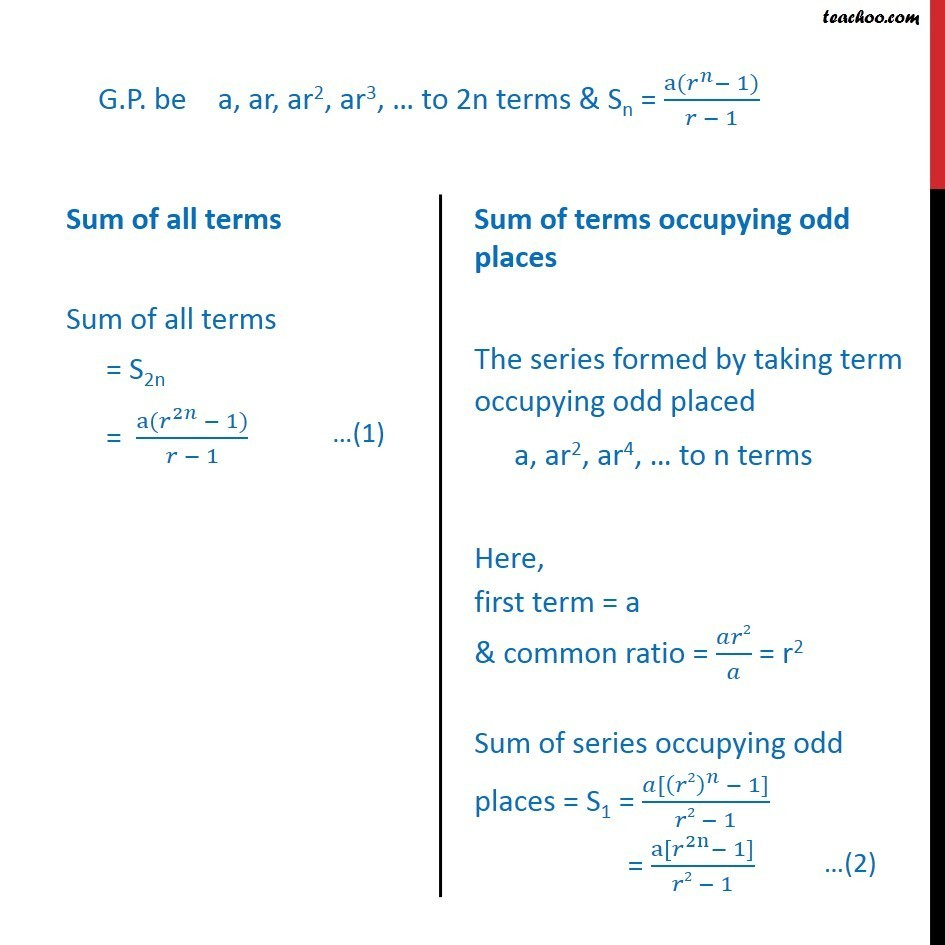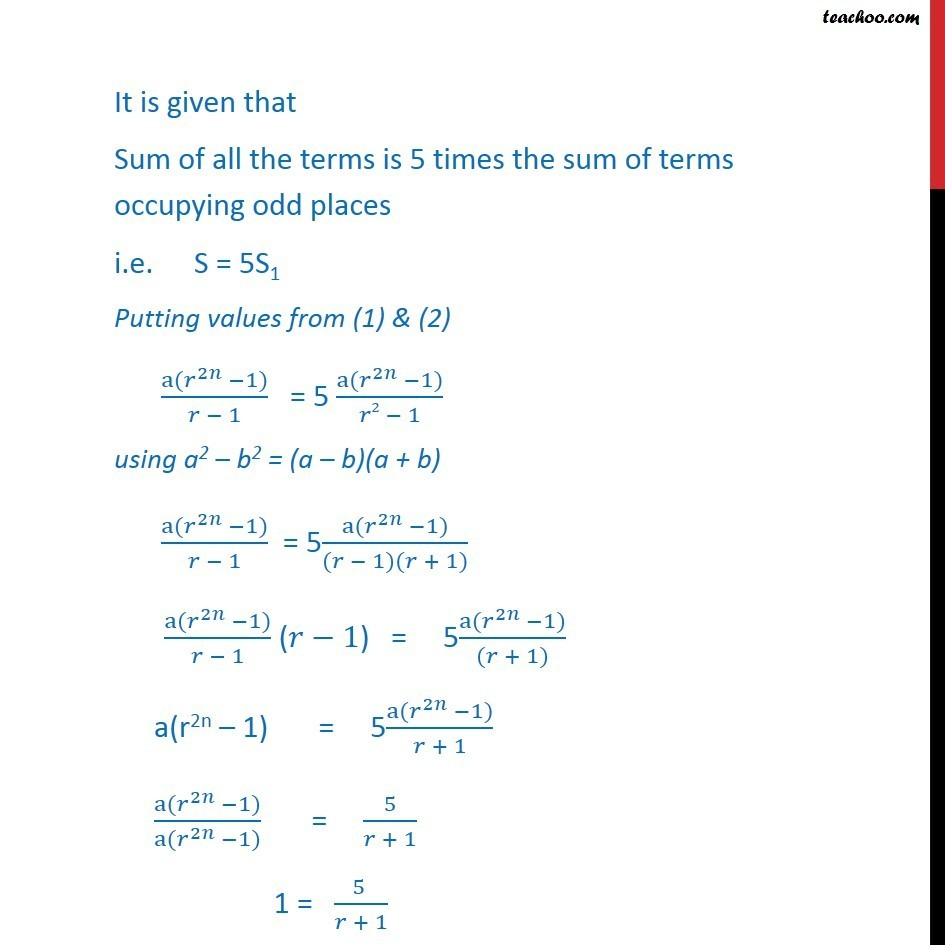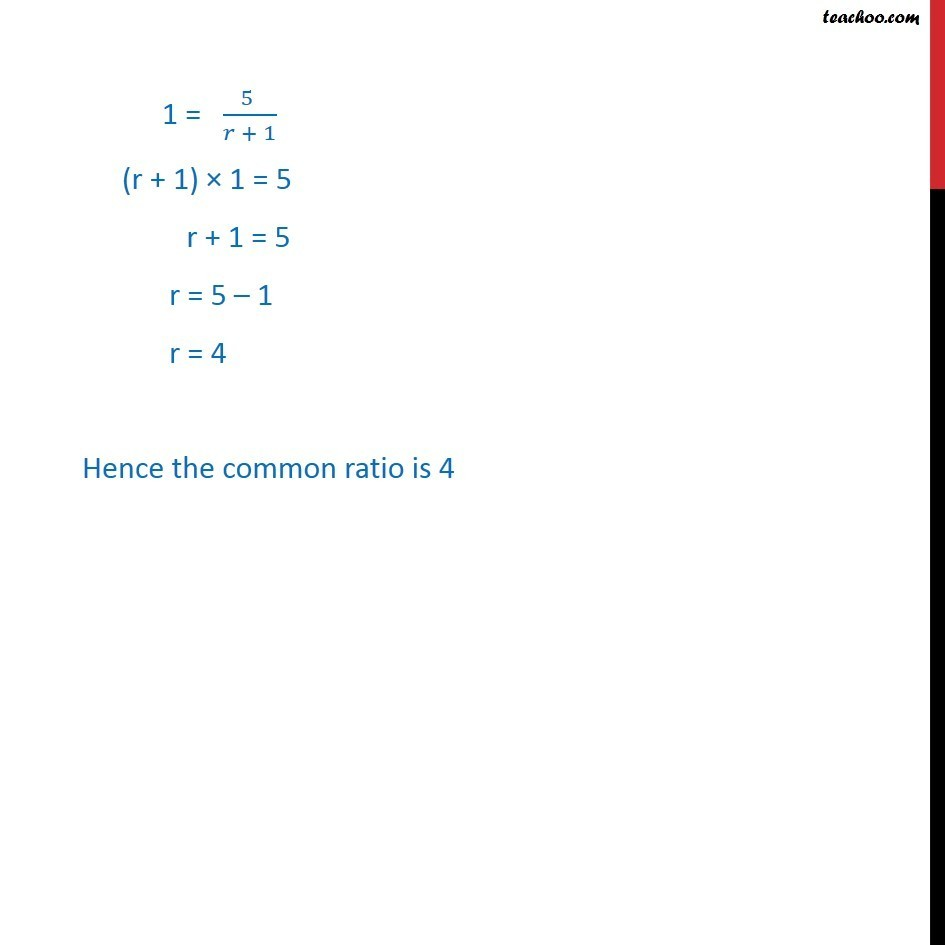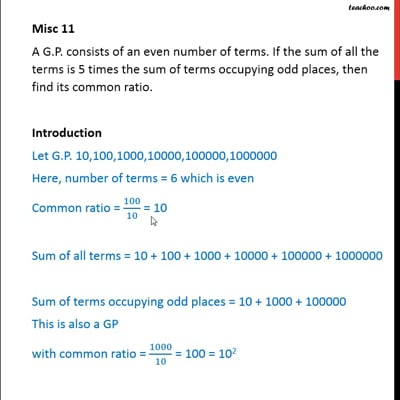This video is only available for Teachoo black users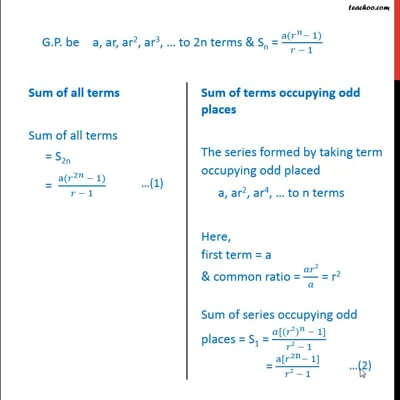This video is only available for Teachoo black users

Solve all your doubts with Teachoo Black (new monthly pack available now!)

### Transcript

Misc 11 A G.P. consists of an even number of terms. If the sum of all the terms is 5 times the sum of terms occupying odd places, then find its common ratio. Introduction Let G.P. 10,100,1000,10000,100000,1000000 Here, number of terms = 6 which is even Common ratio = 100/10 = 10 Sum of all terms = 10 + 100 + 1000 + 10000 + 100000 + 1000000 Sum of terms occupying odd places = 10 + 1000 + 100000 This is also a GP with common ratio = 1000/10 = 100 = 102 Misc 11 A G.P. consists of an even number of terms. If the sum of all the terms is 5 times the sum of terms occupying odd places, then find its common ratio. We know that Sn = (a(𝑟^𝑛− 1))/(𝑟 − 1) Since GP has even number of terms, We take number of terms as 2n (so that it is always even) Let G.P. be a, ar, ar2, ar3, … to 2n terms Now, finding sum of all terms & sum of terms occupying odd places G.P. be a, ar, ar2, ar3, … to 2n terms & Sn = (a(𝑟^𝑛− 1))/(𝑟 − 1) It is given that Sum of all the terms is 5 times the sum of terms occupying odd places i.e. S = 5S1 Putting values from (1) & (2) (a(𝑟^2𝑛 −1))/(𝑟 − 1) = 5 (a(𝑟^2𝑛 −1))/(𝑟2 − 1) using a2 – b2 = (a – b)(a + b) (a(𝑟^2𝑛 −1))/(𝑟 − 1) = 5(a(𝑟^2𝑛 −1))/((𝑟 − 1)(𝑟 + 1)) (a(𝑟^2𝑛 −1))/(𝑟 − 1) (𝑟−1) = 5(a(𝑟^2𝑛 −1))/((𝑟 + 1)) a(r2n – 1) = 5(a(𝑟^2𝑛 −1))/(𝑟 + 1) (a(𝑟^2𝑛 −1))/(a(𝑟^2𝑛 −1)) = 5/(𝑟 + 1) 1 = (5 )/(𝑟 + 1) 1 = (5 )/(𝑟 + 1) (r + 1) × 1 = 5 r + 1 = 5 r = 5 – 1 r = 4 Hence the common ratio is 4